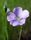## Hash with many variables

I have a join of 2 datasets that takes 1h36 to process, and I thought to reduce the processing time to use hash tables.
Of these tables, one has 1,672,529 rows and 11 variables, the other has 1,443,057 rows and 42 variables.
I'm wondering, in a hash step, which of the 2 tables should be loaded into the hash table and which one to put into the set. Thank you

7 REPLIES 7

## Re: Hash with many variables

The boring but correct answer, is that there is no telling for sure. It depends on a lot of factors. Simply try it.

There is a technique to read many variables into the hash object in the article below. Should save you some typing.

Read Many Variables Into the SAS Hash Object

## Re: Hash with many variables

How large are the tables? How much memory can a sas session use? Are you using sql or a data step now? Are the tables indexed?LinusH
Tourmaline | Level 20

## Re: Hash with many variables

I doubt that you would gain much by jopining two tables in the about the same size.

Can you share the current SQL log, ideally using

``options fullstimer msglevel=i;``

If possible, inrease your settings for MEMSIZE and SORTSIZE.

Data never sleeps

## Re: Hash with many variables

@mariopellegrini wrote:

I have a join of 2 datasets that takes 1h36 to process, and I thought to reduce the processing time to use hash tables.
Of these tables, one has 1,672,529 rows and 11 variables, the other has 1,443,057 rows and 42 variables.
I'm wondering, in a hash step, which of the 2 tables should be loaded into the hash table and which one to put into the set. Thank you

To use the hash object the whole table must fit into memory. The volumes of your two tables look like this could be an issue.

You would normally load the lower volume table into the hash or if there is a one to many relationship then eventually the table that's "one" so you don't need to loop over the hash.

1h36 for the volumes you shared feels "excessive". Are you sure you don't have some many to many join condition? How many rows does your target table have?

Can you please share your SQL code as well as the log with the options as "requested" by @LinusH . Eventually also add option _method to the SQL so we understand how the join executes.

What also would help is a Proc Contents of your two source tables so we can see the size and if there are any indexes or sorts. And what also would help is to understand the relationships between the tables.AhmedAl_Attar
Rhodochrosite | Level 12

## Re: Hash with many variables

To join two wide tables using Hash is possible without loading the entire columns into Hash 😉

Check out this paper HASH + POINT = KEY  by @hashman

Paul illustrates a technique that allows to join two table without put straining your SAS's allocated memory

Hope this helps,

Ahmed

## Re: Hash with many variables

If you know your data well enough, you might find a way to save a lot of memory usage by using multiple hashes for one of your datasets.

Let's say you have dataset A with 11 vars (ID and X1-X10) and 1.6m rows.  And maybe you know that there is a limited number of combinations of variables X7-X10 (let's assume 5,000).  Then you could dynamically set a code for each of those combinations while the hash object is in memory, and recover those codes after the join.

Assuming you are joining B and A on a single variable, (ID), then it might look something like this:

``````data want (drop=_:);
set dummy a (in=ina) b (in=inb) ;
if _n_=1 then do;
declare hash AR (dataset:'dummy (drop=x7-x10)');
/*AR = "A Reduced" - holds ID, X1-X6 and _CODE*/
AR.definekey('id');
AR.definedata(all:'Y');
AR.definedone();

declare hash CH (dataset:'dummy(keep=_code x7-x10)');
/*Holds _CODE, X7-X10*/
CH.definekey('_code');
CH.definedata(all:'Y');
CH.definedone();

/* Edit: added Reverse_CH hash object */
declare Reverse_CH (dataset:'dummy(keep=_code x7-x10)');
Reverse_CH.definekey('X7','X8','X9','X10');
Reverse_CH.definedata('_code');
Reverse_CH.definedone();
end;
if ina then do;  /*Get the _CODE, otherwise Update CH and Reverse_CH */
if Reverse_CH.find()^=0 then do;  /*This is a new combo of X7-X10*/
_code=Reverse_CH.num_items+1;
end;
end;
if inb=1 and AR.find()=0;
CH.find();
run;``````

So instead of having a hash object with ALL of A's data  (1.6m "rows" and 11 vars), you would have three hash objects, one (AR) with 1.6m "rows" and only 8 vars (about a 27% reduction in data values) and the other two (CH and Reverse_CH) with 5,000 rows and 5 vars each.  The latter takes almost no memory at all compared to the savings achieved in the former.

And you could use this technique for additional subsets of variables in A if their combination also has a low cardinality.  You'd just have a code hash for each variable collection.

Edit note: the original post did not include the Reverse_CH hash object instantiation and use.  It's now there.

--------------------------
The hash OUTPUT method will overwrite a SAS data set, but not append. That can be costly. Consider voting for Add a HASH object method which would append a hash object to an existing SAS data set

Would enabling PROC SORT to simultaneously output multiple datasets be useful? Then vote for
Allow PROC SORT to output multiple datasets

--------------------------

## Re: Hash with many variables

We need to see the complete log of the step that does the job (or the steps, if it's done with SORT and MERGE).

Discussion stats
• 7 replies
• 335 views
• 7 likes
• 8 in conversation